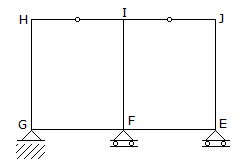# Civil Engineering - GATE Exam Questions - Discussion

### Discussion :: GATE Exam Questions - Section 3 (Q.No.32)

32.

The degree of static indeterminacy of the rigid frame having two internal hinges as shown in the figure below, is[A]. 8 [B]. 7 [C]. 6 [D]. 5

Explanation:

No answer description available for this question.

 Ajinkya said: (Aug 15, 2016) 4 - 3 + 3 * 2 - 2 = 5.

 Malatesh S Patil said: (Jul 28, 2017) @Ajinkya. Can you please elaborate the answer?

 Poobathy said: (Sep 13, 2017) Dsi=4-3=1. Dse=3*2=6. Ds=1+6-2=5.

 Sna said: (Sep 22, 2018) 3 * 9 + 4 - 3 * 8 - 2 = 5.

 Muhammad Zaid said: (Oct 4, 2018) (Total unkown)-(equilb eq). (3m+r)-(3j+a). a=factor use for internal hinge.

 Puja said: (Jan 30, 2019) In loop method, Dse=R-3. Where R is External Reaction..Dsi = 3*No of close loop - Additional condition of equilibrium (members-1). Dse=3+1+1-3=1. Dsi = 3 * 2-(m-1)-(m-1) = 6-(2-1)-(2-1)=4 . Hinge is placed in between 2member so (m-1) is (2-1).

 Prenita said: (Mar 11, 2019) 3*7 + 4-3 * 6-2 = 5.

 Prenit said: (Mar 11, 2019) Thank you all for explaining.

 Vikasgowda C J said: (Aug 2, 2020) Ds=Dse+Dsi. Dse= no.Of reactions(r) - no.of equilibrium equn(s) Dse= 4 - 3. Dse= 1 Dsi= 3( no of closed-loop in a structure) -releases( when a hinge is present between members) i.e, if the hinge is present between 2 members= m-1=2-1=1. Dsi = 3C-1-1= 3(2)-2 = 6 - 2 = 4. Ds = 1 + 4 = 5.

 Mumtaz Ali said: (Nov 18, 2020) Ds = Dsi + Dse = 4 + 1= 5.